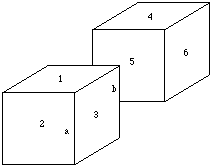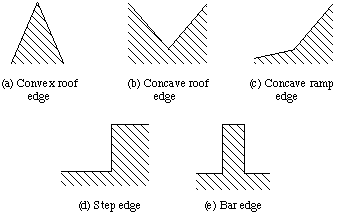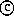Next: Assumptions Up: Line Labelling Previous: Line Labelling

## Introduction

Line Labelling is applicable when two-dimensional lines have been extracted from a two-dimensional image.

Initially, we shall restrict ourselves to polyhedral objects in our scene, i.e. have flat faces bounded by straight edges.

Under this assumption it becomes possible to interpret the edges

• to determine which edges bound which faces, and
• to deduce certain relationships between faces.

Consider the line drawing shown in Fig. 38.Fig. 38 Line drawing of two cubes

It can be seen that

• the edges bound the numbered faces, and that faces 1, 2 and 3 belong to one cube,
• while faces 4, 5 and 6 belong to another (assuming that the two cubes are not joined by a thin sliver that cannot be seen).

An important observation is that

• Every three-dimensional edge is associated with exactly two faces -- one on either side of it,
• A few rather ill-conditioned objects called non-manifold objects are exceptions -- we shall not consider these further here.

Sometimes both of these faces can be seen from a given viewpoint (as in the case of line a in Fig. 38).

If only one of the faces is visible -- the edge is called an occluding edge. On the other side of an occluding edge, the background or a non-touching face is visible.

The basis of the line labelling method is to attempt to classify each edge in the image as being one of:

• a concave edge,
• a convex edge,
• an occluding edge.

Edges of each type are shown in Fig. 39.Fig. 39 Types of edge in a line drawing

Where edges meet only certain possible interpretations of the meeting lines are mutually consistent.

This reduces the number of possibilities for the classification of each edge.

If a line corresponds to an occluding edge:

• It is marked with an arrow. The orientation of the arrow gives the side of the edge on which the visible face lies, using the convention that the visible face lies on the right hand side of the edge, looking along the direction of the arrow.
• Lines corresponding to convex edges are marked with a `+' symbol.
• Lines corresponding to concave edges are marked with a `-' symbol.
• Each edge in the image thus has one of four possible labellings.Next: Assumptions Up: Line Labelling Previous: Line LabellingDavid Marshall 1994-1997